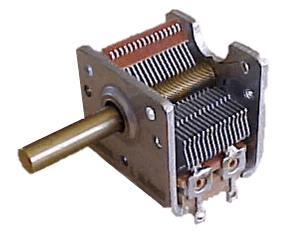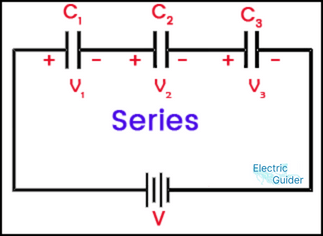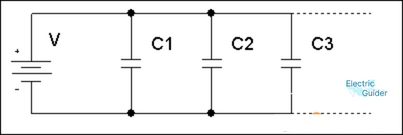# What is a Capacitor ? Types, Principle, Uses

## What is a capacitor?

Capacitor is a passive element that stores energy in the form of electrical charge. Like a small rechargeable battery, capacitor is charged and discharged in a very short time. Capacitor is called capacitor in Hindi and it is called also called condenser.

The property of a capacitor to store electricity is called capacitance. The unit of capacitance is Farad.### Definition of capacitor

If any two metallic plates or layers are separated by the dielectric of an insulating material, it becomes a capacitor. Capacitor is a passive component that stores and discharges electrical energy by charging it.

## Different types of capacitors and their applications

Capacitors are mainly divided into two classes

(i) Fixed Capacitor- The value of this type of capacitor remains the same without changing while in use.

(ii) Variable Capacitors – Capacitors whose value can be changed while in use.

According to the dielectric used in the capacitor, the name of the capacitor is given, such as air capacitor, mica capacitor, paper capacitor, ceramic capacitor, plastic capacitor etc.

#### (a) Air Capacitor

In this type of capacitor, the air between the plates acts as dielectric instead of dielectric. These types of capacitors are often made variable, in which the aluminum plates inside each other increase the capacitance.

The change in capacitance is made linear or non-linear. This capacitor is shown in the figure. In a reversible capacitor, one plate or plates is stationary and the other plate or plates are movable. The capacitance increases as the plates move in and the capacitance decreases when the plates come out.Application

• oscillator
• in test equipment.

#### (b) Mica Capacitor

The plates of this capacitor are made of tin foils or tin sheets. Metal plates are made in two sets in multiple layers. These capacitors are made of both fixed and variable type. Trimmer, Pedder capacitors are made of variable mica type. These are made in the 5 pF to 10 uF range. The frequency range of these capacitors is up to 10 MHz.Application

• To do tuning as a trimmer, padder.

#### (c) Paper Capacitor

Kraft paper impregnated with wax, resin is used as dielectric in this type of capacitor.

Capacitors are made of two types. In the first type, the sheet of paper is coated on only one side and in the second type, the metallic coating is done on both the sides of the sheet of paper. After wrapping the layers, the capacitor is dipped in wax and covered with a plastic or metal cover.

The range of these capacitors is from 1000 pF to 1 uF, and the voltage range of these capacitors is from 3000 to 1500V.Application

• 1 phase in permanent driven motor
• Power Coefficient Improvement (PF improvement)
• RF bypass
• For coupling.
• blocking.
• D as coupling capacitor.

#### (d) Ceramic Capacitor

It is made from a product of steatite ceramics which has a high electronegativity. It is made by grinding it, adding binda, pressing it into shape and cooking it at 1400°C. These are made in various pearl, disc rectangular, pinup, tubular shapes. Their range is 5 mF to 10 uF and the voltage rating is 60V to 10 kV.Application

• Medium wave transmitter.
• in military equipment.
• High quality (Q) circuit.
• In high power amplifier.

#### (e) Electrolytic Capacitor

This type of capacitor uses dielectrics from a very thin layer of aluminum or tantalum oxide. They are made of semi-polarized and non-polarized types. go. is the symbol for polarized capacitor and denotes unpolarized.

The capacitance range of electrolytic capacitor is 1 to 2000 uF, voltage range from 1 to 50 V and temperature coefficient is 200 to 500 PPM/°C.
It is marked (-), the short lead is used to identify the (-) terminal. It gets damaged if the voltage exceeds the marked voltage.Application

• In power supply filter circuit
• In bypass circuit
• Amplifier circuits
• For coupling

## Capacitor and its principleCapacitor is an arrangement in which dielectric insulating material is placed between two conductors to store electrical energy and take away this energy when needed.

It mainly consists of conductor and insulating material. These conductors are metals like tin, aluminum etc. Insulating materials are mica, ebonite, air etc. The two plates are parallel to each other with an insulating material placed between them. Due to this insulating material, both the conductors or the plates are not able to meet with each other. Terminals are removed from both ends.

Two plants are shown in parallel, in which a switch and a battery are also attached. The positive end of the battery is attached to one plate A and the other end to another plate B.

When the switch s is turned on, a momentary flow of current occurs in the circuit, which charges the capacitor. Plate A positive and plate B negative. The negative end of the battery supplies electrons to plate B and charges it negatively. Due to electrostatic induction action in the capacitor, plate B repels some of the electrons of plate A and makes it positively charged. These repelled electrons are attracted by the positive end of the battery. Thus, a momentary flow of electrons from the negative end of the battery to the positive end causes the capacitor to charge.

A potential difference (p.d.) is developed between the plates of the capacitor. This potential difference takes some time to reach its peak value and in this time the charging current reaches zero from its peak value. If the capacitor is now isolated from the battery and its plates are ‘short-circuited’ with a wire, an electric spark is produced. This spark verifies that charge was present in the capacitor. Once the capacitor is charged, we see that

(i) The transfer of electrons from plate A to plate B through the circuit results in the accumulation of charge in the capacitor.

(ii) Only momentary current flows in the circuit but DC. cannot flow continuously in the circuit.

(iii) As long as charge is present in the capacitor, its dielectric remains with strain.

(iv) the maximum kilovolt value for a dielectric per millimeter of thickness that it can safely bear. Its dielectric strength is called.

## Types of Capacitors and Their Uses

On the basis of value control, capacitors are mainly of the following two types:

1. Fixed Value Capacitor
2. Variable Capacitor

1. Fixed-Value Capacitor: A capacitor whose capacitance remains constant is called a fixed-value capacitor. On the basis of the dielectric used in it, they are of the following types

(a) Paper Capacitor
(b) Mica Capacitor
(c) Electrolytic Capacitor
(d) Ceramic Capacitor

### (a) Paper Capacitors

It consists of two long and thin strips of tin or aluminum which are alternated between three strips of waxy paper. All the strips are wrapped and given the shape of a cylinder. This is taken care of while wrapping. that one conducting strip does not touch the other conducting strip. This cylinder is either covered with bitumen material or kept in a cylindrical aluminum vessel. One connecting lead each is attached to both the driving strips.### (b) mica capacitor

In this, many fine conducting plates are kept separate from each other by mica sheets. The plates are connected alternately to the two connecting leads. Plate 1,3,5…..etc from one lead and plate 2.4, 6….etc from other lead. This distance arrangement is covered with bitumen material and given the form of rectangular solid.### (c) Electrolytic Capacitor

In this, a set of aluminum plates is kept in an aluminum vessel immersed in a solution of aluminum borate or sodium phosphate. These capacitors are used only in DC circuits because when the plates are connected to DC. A chemical reaction takes place in the solution when it is supplied from the positive end of the supply and from the negative end of the supply. As a result of this action, a thin layer of aluminum oxide is formed on the aluminum plates, which acts as a dielectric between the plates and the container (or solution).

### (d) ceramic capacitor

It consists of two plates of aluminum which are separated from each other by a layer of ceramic compound. It is the smallest capacitor of all types of capacitors. Its capacitance value ranges from 5pF to 0.1 uF and is used in electronic circuits.

2.Variable Capacitor: The capacitor whose capacitance value can be changed in a predetermined value range is called variable value capacitor.

It consists of two groups of plates made of aluminum which are called ‘rotor’ and ‘stator’. The air between the plates acts as a dielectric. When the rotor plates are completely covered by the stator plates, the value of capacitance is maximum. In contrast, when the rotor-plates are completely outside the stator-plates, the capacitance value is minimum.
These capacitors are used in receivers, transmitters, etc. and are known as air gang capacitors or gang capacitors.## Electrical Uses of Capacitors

1. In Single Phase Motor: Capacitor is used to divide a single phase into two parts in a single phase motor, like in the motor of a ceiling fan and a table fan.
2. In fluorescent tubes: In the starter of fluorescent tubes, capacitors are used to start the tubes. In addition, a capacitor is used in parallel with the tube. So that the tube does not interfere with the reception of nearby receivers etc.
3. In inductive circuits: In all types of inductive circuits, capacitors are used to improve the value of power factor.
4. In distributors: Capacitors are used to reduce sparking in distributors of auto vehicles, such as in petrol operated cars, buses, trucks etc.

The capacity of the condenser depends on the following factors

(a) directly proportional to the area of ​​the plates.

(b) Inversely proportional to the distance between the plates.

(c) on the nature of the insulation between the plates (Dielectric constant)

## How to connection capacitors

Capacitors can be applied in a circuit in two ways.

• Series Connection
• Parallel Connection

### Series Combination of Capacitors

If the negative (-) end of one capacitor is connected to the positive (+) end of the second capacitor and the other negative end to the positive terminal of the third capacitor, then it is called a series combination. In this combination all capacitors have the same charge (q).If n capacitors are connected in series thenIn other words,

When two or more capacitors are connected directly end to end, it becomes a series connection. In this, the charge of each capacitor is same but their voltage is different.

V=V+V2+V3

Equivalent capacitance is less than minimum capacitance.

### Parallel Combination of Capacitors

When one of the positive ends of each capacitor is connected to the positive terminal of the source or supply and the other negative ends to the negative terminal of the supply, the capacitors are said to be connected in parallel.In other words,

When two or more capacitors are connected in such a way that their positive-plates are at one place and the negative-plates at another, they become parallel connection.
In parallel series, all capacitors have the same potential difference and different charge.

If n capacitors are connected in parallel, then

C=C1+C2+ C3………..

## What is capacitance

The property of a capacitor to store electricity is called capacitance or the amount of charge required to produce a unit potential difference between the plates. When a coulomb of charge is placed on any one of the two plates of a capacitor, a potential difference of V volt is established between the plates.The unit of capacitance is Farad, it is also denoted by coulomb per volt (coulomb/volt).

The capacitance of a Farad capacitor is one in which a potential difference of one volt is required for a coulomb of electric charge.

## Capacitor charge and discharge

### Charging of a capacitor

As shown in the figure, the circuit in which the resistance R is connected in series with the capacitor C and the constant DC voltage V is applied in the side of the circuit. When the switch S is brought to terminal 1, the potential difference across the capacitor becomes zero but eventually increases to equal to the supply voltage V. While increasing the voltage, the capacitor collects the energy taken from the supply in the form of static electrical energy. Let at any instant the voltage across the side of the capacitor be V volts and the charge collected in the capacitance is q coulomb.

### Discharging of a capacitor

Let the voltage across the capacitor at present be V. In immersion the charge of the capacitor starts decreasing and the current starts flowing in the opposite direction. Let the instantaneous value of the current be . And there is an instantaneous charge of the capacitor. Since by bringing the switch to point 2, the supply connection with the circuit is severed.

## How to check capacitor

If on checking the continuity of the capacitor, the needle of the meter gives no deflection, that means maximum resistance is observed. So the capacitor may be right and it may also be open. Because neither the right capacitor passes dc nor the open capacitor.

SHOTING CONDITION:- If the meter needle shows full deflection on checking the continuity of the capacitor with the meter. That is, if the meter needle comes to zero from left to right, the capacitor is shorted.